Courses

# Chapter Notes - Force & Pressure Class 8 Notes | EduRev

## Class 8 : Chapter Notes - Force & Pressure Class 8 Notes | EduRev

The document Chapter Notes - Force & Pressure Class 8 Notes | EduRev is a part of the Class 8 Course Science Class 8.
All you need of Class 8 at this link: Class 8

What is Force?

A pull or push over an object is called force.

Example:

• To open a drawer, one has to pull it; and to close the drawer one has to push it. Thus, in both conditions, a person applies force by pull or push.
• To kick a ball, one has to push it, i.e. a force is applied.
• To ride a bicycle one has to push the pedal. In this condition, force is applied while pushing the pedal.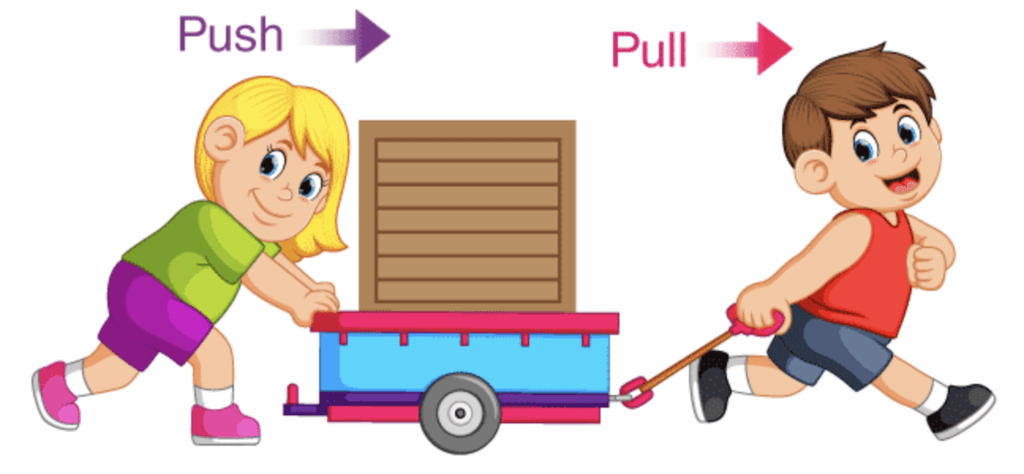Forces are due to Interaction:

To apply a force over an object interaction between object and source of force is necessary.

Example:

• To open a drawer one has to pull by holding it, drawer will not open otherwise.
• To kick a ball one has to touch it, otherwise, force will not be applied over the ball.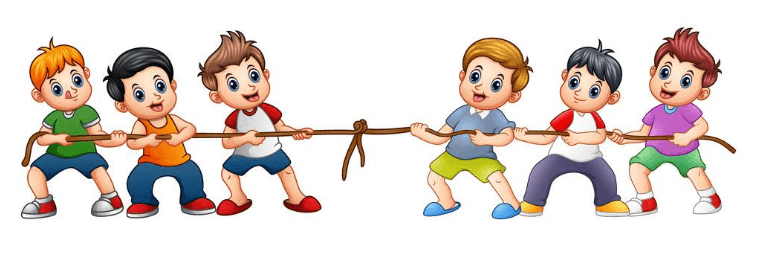Force due to Interaction

Exploring Forces

• The measure of the amount of strength of the force is called the magnitude of force. Thus, strength or amount of force is expressed in terms of magnitude.
• Two or more forces on the same object can be applied in the same direction and opposite direction.

1. Forces applied in the Same Direction

When more than one force is applied in the same direction, the total force is the addition of magnitude of both the forces.

Example: It is difficult to push a car by a single person, but two or more persons can collectively push the same car easily. This is because when more than one person tries to push the car, magnitudes of forces applied by each of the people are summed up and applied over than car, and car is pushed easily because of the greater magnitude of force.

That means if three units of force are applied over an object by each of the two persons,

The total unit of force = sum of both forces = 3 unit + 3 unit = 6 unit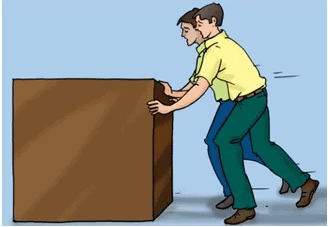Force applied to the Same Direction

2. Forces applied in Opposite Direction

When two forces are applied over an object from opposite directions, the total effective force is the difference between the two forces.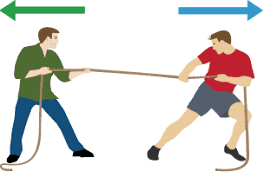Force applied to Opposite Direction

Example:

• When two persons are pushing a box in opposite directions and the magnitude of both the forces is equal, then box will not move, because the difference of two forces will be equal to zero.
Let a person applying 4 unit of force in one direction and the other person is also applying 4 unit of force in opposite direction on the same wooden box.
Therefore, the magnitude of total force = 4 unit – 4 unit = 0
• If one person is applying a force of 4 unit in one direction and another person is applying a force of 6 unit in opposite direction,
Then total magnitude of force = 6 unit – 4 unit = 2 unit
Thus, the force will act in the direction of a larger magnitude of force.

Force can Change the State of Motion

An Object can be in two states.

1. State of Rest
An object is called in the state of rest when it is not moving. This means a stationary object is called in the state of rest.
For example: a building, an electric pole, a ball kept over the ground and not moving, etc.
2. State of Motion
A moving object is called in the state of motion.
For example: a moving car, a moving ball, etc.

A force can speed up a moving object. Force can decrease the speed of a moving object. Force can stop a moving object. Force can change the direction of a moving object. Force can move a stationary object.

Example:

• In the game of cricket, when a batsman pushes a fast-moving ball in the same direction as the movement of the ball, the speed of the ball is increased.
• When a batsman pushes a fast-moving ball in the opposite direction the speed of the ball decreases; because of force being applied from his bat.
• When a player in the game of football kicks the ball to his mate, he changes the direction of the moving football by applying force through his kick.
• A goalkeeper stops the football from going towards the goal post by applying force by his hand.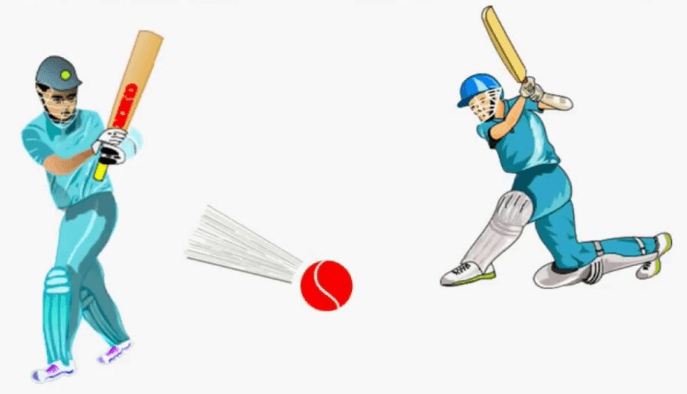Force Changes the State of MotionForce can Change the Shape of an Object

• When you apply force to an inflated balloon; using your hand from both sides, the force of pressure changes the shape of balloon.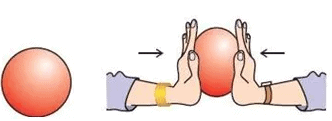• The change in the shape of dough into bread by applying force with a rolling pin.
• A blacksmith changes the shape of an iron rod by applying force using a hammer.
• You can change the shape of the rubber band and spring by stretching it in opposite direction.

Types of Forces

By applying force, one can make an object at rest move. This application of the force can be done either by touching the object or by without touching the object. Therefore, there are two types of forces:

• Contact force
• Non-contact force

Contact Force

Forces that require a touch or contact to be applied are known as contact forces.

Example:

• Muscular forces
• Frictional forces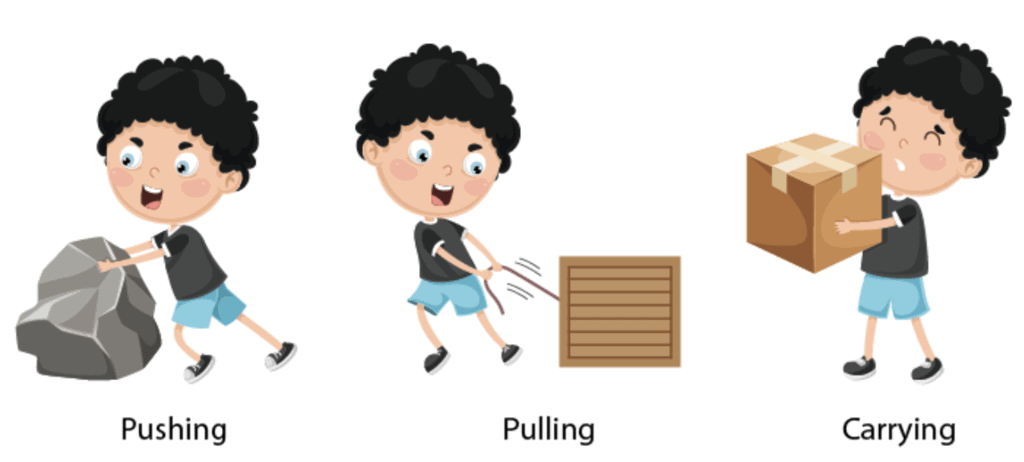Muscular Force

The force applied by the effort of our muscles

Example: lifting a heavy box, pulling a bucket of water, pedalling a cycle.

Frictional Force

Friction is a force exerted by a surface against the motion of a body across its surface.

• Friction is the reason why your car comes to a standstill if you don’t press the accelerator.
• The frictional force is opposing the rotation of the wheels of your car. Therefore, if you leave the accelerator, the friction slowly removes all the force from the car and when it has removed the last bit of force, the car comes to a standstill.
• Another example, if a book slides across the surface of a desk, then the desk exerts a friction force in the opposite direction of its motion causing it to stop sliding after a finite distance. Friction is a type of contact force only.

Non-Contact Force

The force which is applied on the body without coming in contact is known as non-contact force.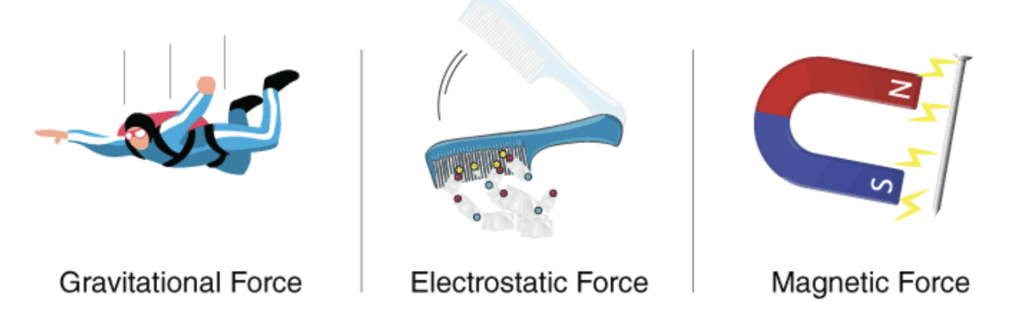Gravitational Force

• The attractive force that a body experience towards the centre of the earth is called the force of gravity due to earth.
• Property of the universe, every object attracts or exerts a force on every other object.

Electrostatic Force

The force of attraction or repulsion experienced by a charged body from another charged body in the same vicinity is known as Electrostatic Force.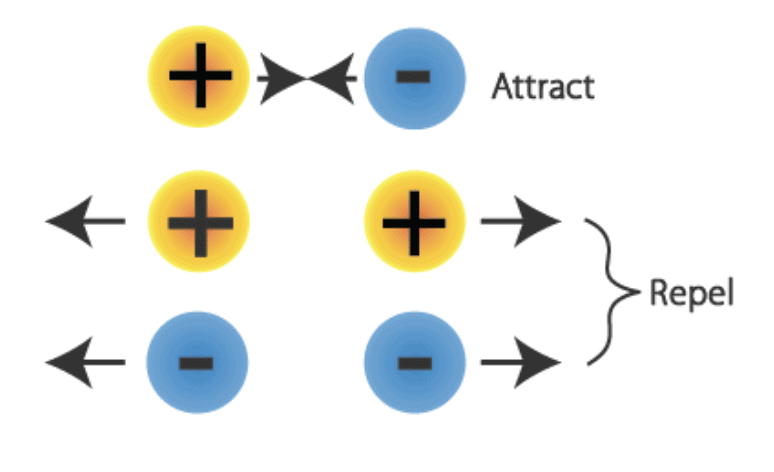Magnetic Force

The magnetic force between two moving charges may be described as the effect exerted upon either charge by a magnetic field created by the other.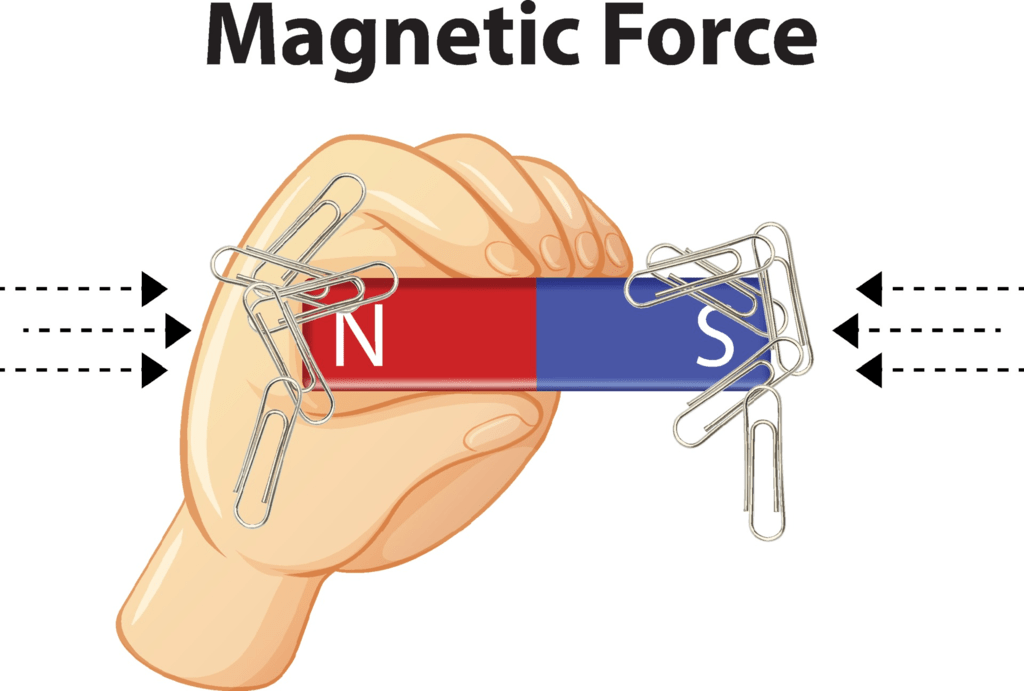What is a Pressure?

Pressure is defined as the force per unit area. If a force F is applied on a surface of area A, then the pressure P is defined as: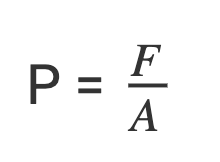Distribution of pressure

Force acting on a smaller area applies more pressure than the same force acting on a larger area.

Examples:

• Porters place around cloth on their heads to increase surface area and reduce pressure.
• A sharp knife cuts better as more pressure is exerted over a smaller area.

Pressure in fluids

The pressure exerted by a fluid(gases or liquids) in a container is transmitted undiminished in all direction on the walls of the container.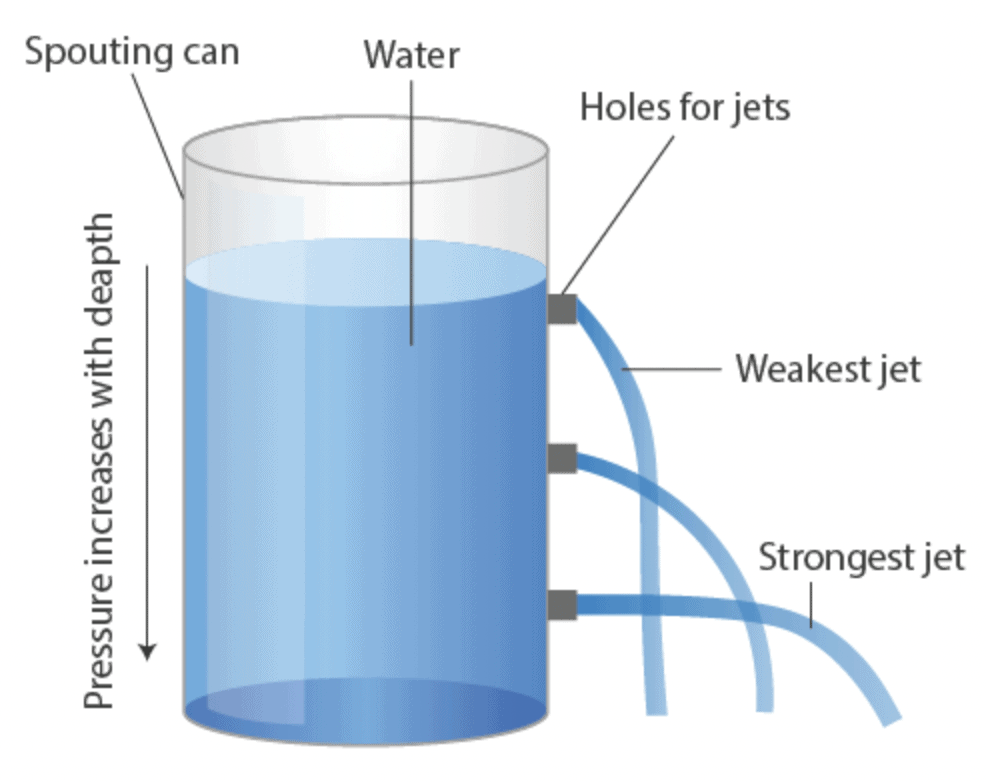Upthrust

The upward force exerted by a fluid on an object is known as upthrust or buoyant force.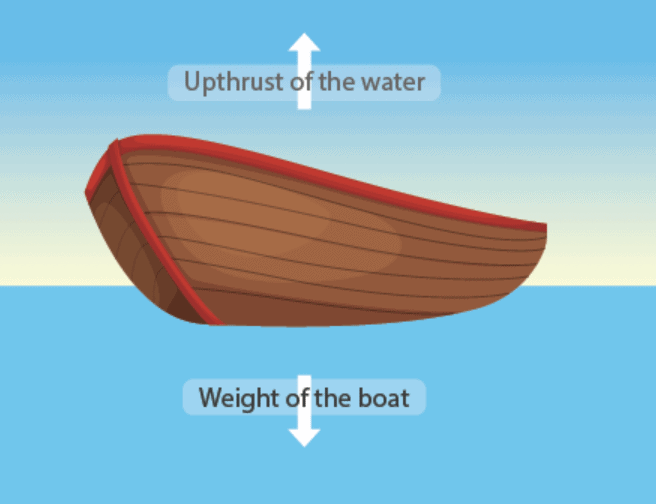Gaseous pressure

Gases exert the same pressure on the walls of the container in all directions.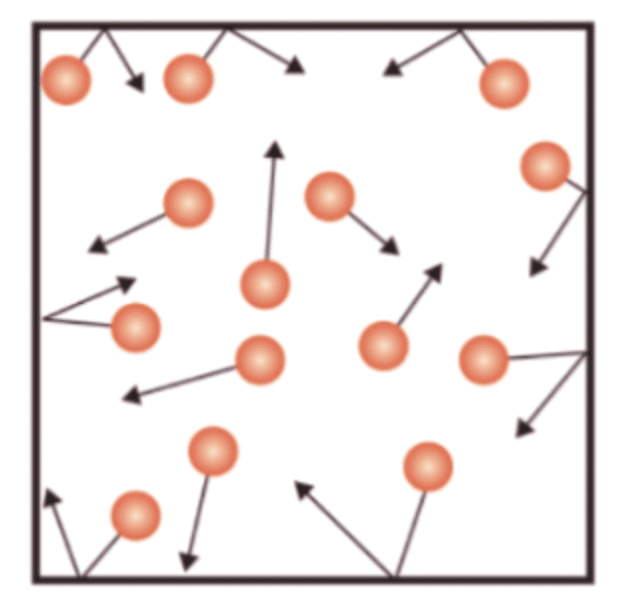Atmospheric pressure
• Our atmosphere extends to several kilometres above sea level. The weight of the air acts as pressure known as atmospheric pressure.
• The pressure in our body balances the atmospheric pressure and that is why we do not feel it.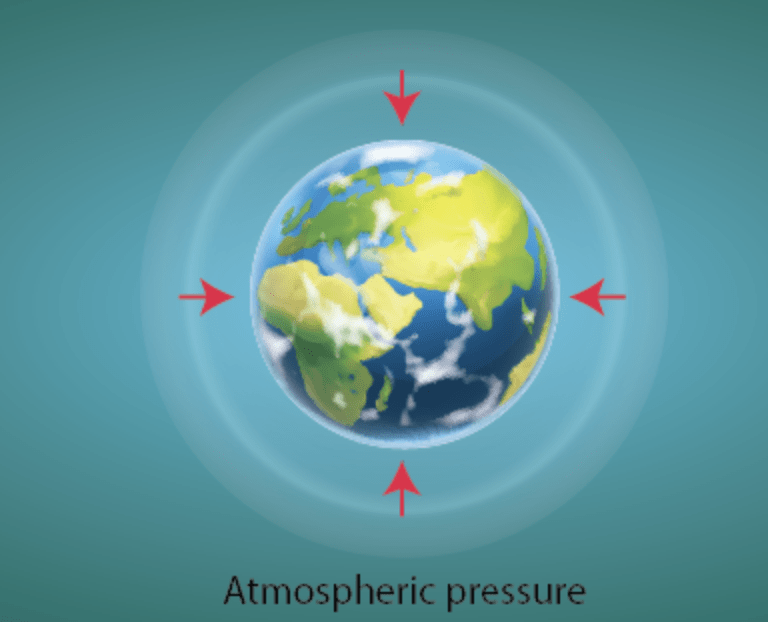Offer running on EduRev: Apply code STAYHOME200 to get INR 200 off on our premium plan EduRev Infinity!

## Science Class 8

143 videos|244 docs|81 tests

,

,

,

,

,

,

,

,

,

,

,

,

,

,

,

,

,

,

,

,

,

;